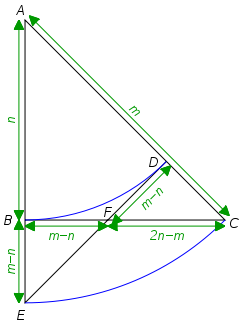# All the Way DownCaltech number theorist Tom Apostol devised this elegant proof of the irrationality of$\sqrt{2}$.

Suppose the number is rational. Then there must be an isosceles right triangle with minimum integer sides (here, triangle ABC with sides n and hypotenuse m).

By drawing two arcs as shown, we can immediately establish triangle FDC — a smaller isosceles right triangle with integer sides.

This leads to an infinite descent. Hence n and m can’t both be integers, and$\sqrt{2}$ is irrational.